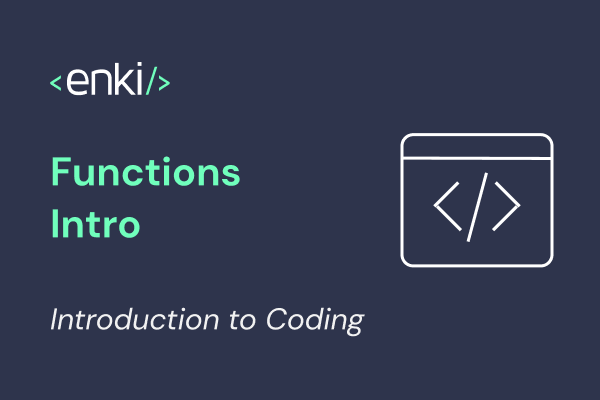This is part of “An intro to Coding” series of posts, with content from the Enki app. If you stumbled upon this, you can start from the beginning.

Functions allow us to group commands and create new ones.

Treating a function as a command means to give it some data and ask it to produce a result.

This is commonly called *running* the function.

The terms calling, executing and invoking the function are also used. All of them mean the same thing.

As an example, imagine that you had a function called multiplyTwoNumbers.

Then you run it, you would give it the numbers 5 and 3 and it would return 15 to you as the result.

We can think of a function as divide into 3 parts.

• input: the data given to the function
• body: the commands (instructions) performed within the function
• output: the result sent out of a function

💡 The inputs to a function are also called arguments.

💡 The outputing of a result from a function is also called returning a result.

Here's how you can write the multiplyTwoNumbers function using our own made-up¹ language:

To call this function we write its name, followed by a pair of ().

💡 If you take a closer look, the multiply within multiplyTwoNumbers is another function! Functions can call other functions.

In the above code, the output of multiply is saved into a variable called result and then that variable is passed out as the output of multiplyTwoNumbers.